# FIR Filtering Demonstration

## Some initial setup

The code immediately below just sets up a few things that will be used for the demo, including generating the input signal that we will be working with. These lines do not directly relate to the filtering aspect of the demonstration.

In :
from IPython.display import Audio
%run DemoSignals.ipynb
rcParams['figure.figsize'] = 10, 8


## Foreword

This demonstration presents a very contrived scenario that is unlikely to crop up in the real world. I would have demonstrated a more relevant application of filtering (perhaps selecting a radio station, for example), but I instead chose to work with audio signals for a few reasons: - We can directly observe these signals using our sense of hearing - Audio deals with real-valued samples - Audio is already at baseband; no need to worry about mixing down higher frequency signals - Allows us to concentrate on the FIR filtering instead of getting hung up on these other aspects - (...and audio is of personal interest to me)

Despite the contrived nature of this demonstration, there are still analogies that can be drawn to more common filtering applications.

## Problem Statement

Given an audio signal containing three melodies of non-overlapping frequencies, use FIR filtering to isolate each of the melodies.

### Knowns

• Sampling rate = 8kHz
• Melody bands:
• Channel 1: ~146Hz - ~785Hz
• Channel 2: ~932Hz - ~1569Hz
• Channel 3: ~1864Hz - ~3730Hz

First, we will look at using the Window Design Method to generate low pass, bandpass, and high pass filters to filter each of these signals. Then, we will take a quick look at using the Parks-McClellan method to filter the signals.

## The Input Signal

In :
fs = 8000
specgram(input_signal, Fs=fs)
Audio(input_signal, rate=fs)

Out: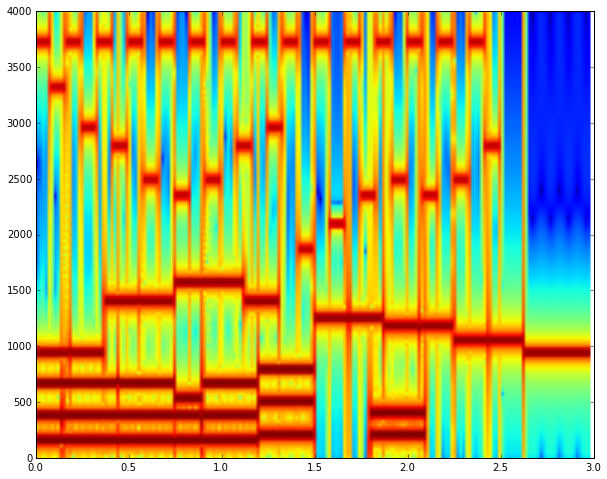## Window Design Method

#### Low Pass Filter

First we'll take a look at creating a low pass filter to pass only the "channel 1" audio.

From above the above list of knowns (and the spectrogram), we see that channel 1 contains frequencies up to around $785\text{Hz}$. Channel 2 starts at around $932\text{Hz}$. This leaves a very narrow transition band of $932-785=147\text{Hz}$, or around $0.018375f_s$. We will need a steep rolloff to accomplish this.

The first step to the window design method is to design an ideal "brick wall" frequency response in the frequency domain. What size FFT should we start with?

• Consider a 64-point FFT
• Each frequency bin would be $8000/64=125\text{Hz}$
• Frequency bins around the transition region include $\{\dots, 750, 875, 1000, \dots\}$
• If we made an ideal passband that includes the $875\text{Hz}$ bin, we would ensure that the desired $785\text{Hz}$ signal is in the passband, but since the stopband doesn't start until \$1000, this means that channel 2's signal is not completely in the stopband
• Consider a 128-point FFT
• Each frequency bin would be $8000/128=62.5\text{Hz}$
• Frequency bins around the transition region include $\{\dots, 750, 812.5, 875, 937.5, 1000, \dots\}$
• Great! We'll make our ideal passband extend up through the $812.5\text{Hz}$ bin and begin our ideal stopband at $875\text{Hz}$. This unambiguously places all of the channel 1 data in the ideal passband and all of the channel 2 data in the ideal stopband
• To create the ideal filter response, then, set $812.5/62.5=13$ bins on either side of DC to 1, and the rest of the bins to 0

Let's give this a try...

In :
N = 128
response = zeros(N)
response[range(-13, 14)] = 1

stem(response);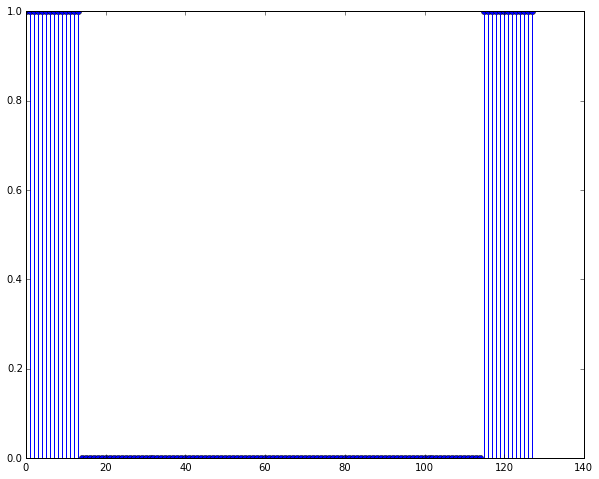Now to get the filter coefficients, take the inverse FFT of the ideal filter response above

In :
coefficients = real(fft.ifft(response)) # Numerical error results in a tiny imaginary part while taking IFFT; discard it
stem(coefficients);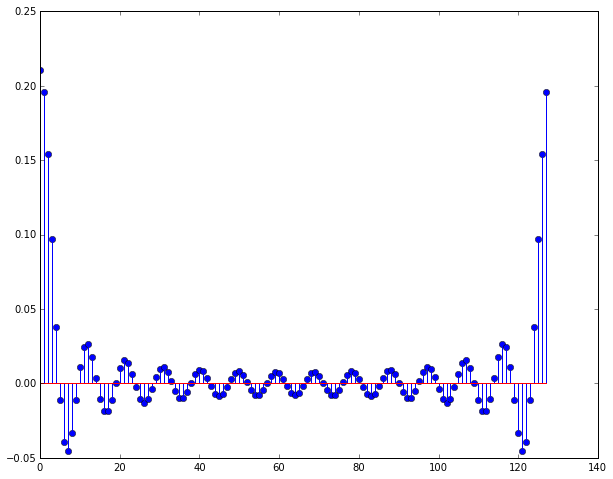We're not quite there. We're interested in the coefficients centered around time $t=0$. Since the IFFT considers the time domain periodic, we simply wrap the signal (see Figure 5-18 in the text).

In :
coefficients = roll(coefficients, N/2-1)
stem(coefficients);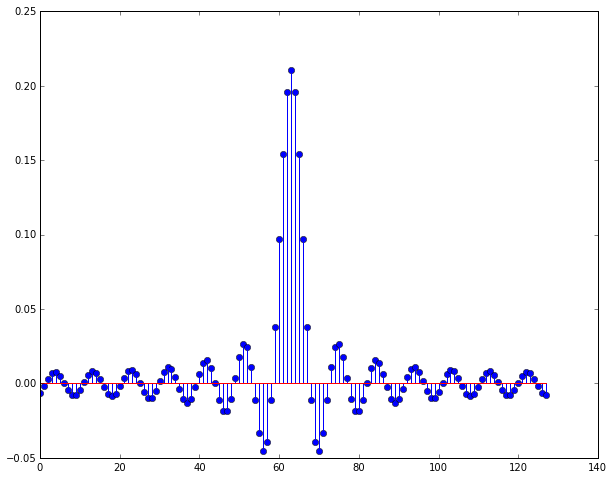We also want to truncate the coefficients so that they're symmetric. Lop off the unmatched 128th coefficient:

In :
coefficients = coefficients[:-1]
stem(coefficients);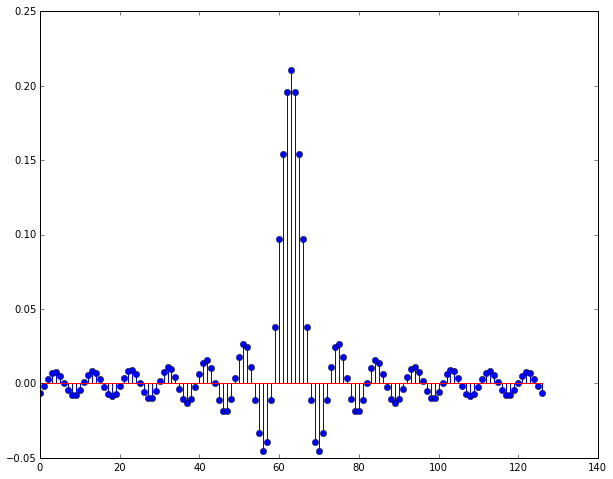We've got our filter coefficients. Now we just need to convolve them with the input signal to get our filtered output. From Equation 5-6 in the text:

$y(n)=\sum_{k=0}^{M-1} h(k)x(n-k)$

Here's a straightforward implementation of the convolution operation (of course, we could just use numpy's convolve() function, or do this in some other more "Pythonic" manner, but let's be explicit for now):

In :
def my_convolution(x, h):
""" Naive convolution implementation

Args:
x: the input signal
h: the filter coefficients
Returns:
the convolution of x and h

"""
M = len(h)
y = []
for n in arange(0, M + len(x) - 1):
y.append(0)
for k in arange(0, M):
if (k < len(h) and (n -k) < len(x)):
y[n] += h[k] * x[n - k]

return y


Cross our fingers and try out our filter:

In :
output_signal = my_convolution(input_signal, coefficients)

specgram(output_signal, Fs=fs)
Audio(output_signal, rate=fs)

Out: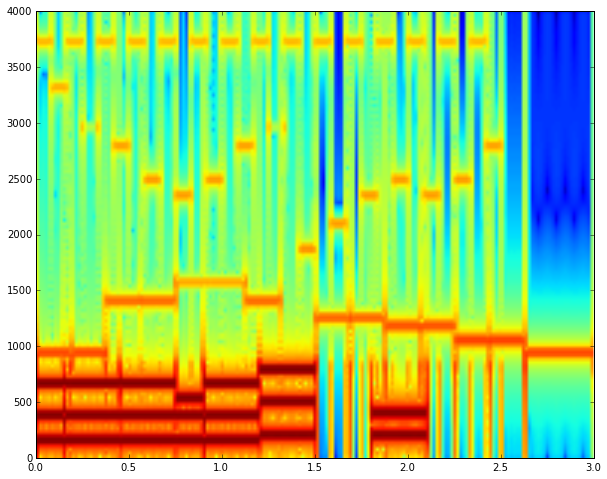#### Results

That filter performed just "okay". We can see from the spectrogram (and we can hear if we listen carefully) that the other channels are still apparent in the signal.

To understand why, let's take a look at the frequency response of the filter that we created. Remember, we can obtain the frequency response of an FIR filter simply by taking the FFT of the filter coefficients.

In :
pad_to = 2048
freq_axis = linspace(0, fs, pad_to, False)

plot(freq_axis, 20 * log10(abs(fft.fft(coefficients, pad_to))));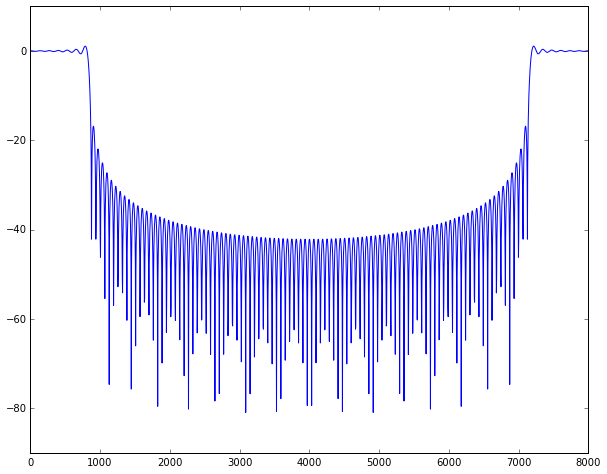#### Windowing

The frequency response above shows us that although we achieved a quick rolloff, the side lobes in the stop band are still relatively high.

Let's see what happens if we apply a windowing function to our filter. We'll use the Blackman window because of it's good side lobe attenuation.

In :
w = blackman(len(coefficients))
coefficients_windowed = w * coefficients

output_signal = my_convolution(input_signal, coefficients_windowed)
specgram(output_signal, Fs=fs)
Audio(output_signal, rate=fs)

Out: